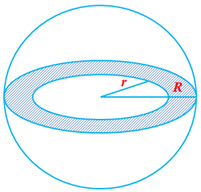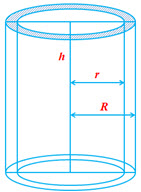Cone

Parameters of a Cone: Radius of the Base ($r$), Height of the Cone ($h$) and Slant Height of a Cone ($l$)Volume of a Cone$= \frac{1}{3} \pi r^2 h$

Curved surface area of a cone$= \pi r l$

Total surface area of a cone = Curved Surface area + Area of the base$= \pi r^2+ \pi r l$

Also note that$l = \sqrt{r^2+h^2}$

SphereParameters of a Sphere: Radius of the Sphere ($r$)

Volume of the sphere$= \frac{4}{3} \pi r ^3$

Surface area of a sphere$= 4 \pi r^2$

HemisphereParameters of a Hemisphere: Radius of the Hemisphere ($r$)

Volume of the hemisphere$= \frac{1}{2} \{\frac{4}{3} \pi r ^3 \} = \frac{2}{3} \pi r^3$

Total surface area of a hemisphere$= \frac{1}{2} \{4 \pi r^2 \} + \pi r^2 = 3 \pi r^2$Spherical Shell (hollow)

Parameters of a shell: Internal Radius of the Shell  ($r$) and External Radius of the Shell ($R$)

Volume of the shell$= \frac{4}{3} \pi (R^3-r^3)$ (this is also the volume of the material used to make the hollow shell)

External Surface are of spherical shell is the same as that of a sphere of radius R$= 4 \pi R^2$Hemispherical Spherical Shell (hollow)

Parameters of a hemispherical shell: Internal Radius of the Shell ($r$) and External Radius of the Shell ($R$)

Volume of the shell$= \frac{2}{3} \pi (R^3-r^3)$ (this is also the volume of the material used to make the hollow shell)

Total surface are of the shell = outside surface area of the shell + Inside surface area of the shell +area of the ring$= \frac{1}{2} (4\pi R^2) + \frac{1}{2} (4\pi r^2) + (\pi R^2 - \pi r^2)$$= 2 \pi (R^2+r^2) + \pi (R^2-r^2) = 3 \pi R^2 + \pi r^2$Cylinder

Parameters of a Cylinder: Radius$(r)$ of the base and Height$(h)$ of the Cylinder.

Area of cross section of a cylinder$= \pi r^2$

Perimeter of the cross section$= 2 \pi r$

Volume of the cylinder$= \pi r ^2 h$

Curved surface area$= 2 \pi r h$

Total surface are of a solid cylinder$= 2 \pi r h + 2 \pi r^2 = 2 \pi r (r + h)$Hollow Cylinder

Parameters of a hollow cylinder: Internal Radius$(r)$, External Radius$(R)$, Height$(h)$

Thickness of the wall of the cylinder$= (R- r)$

Area of the cross section$= \pi R^2 - \pi r^2 = \pi (R^2-r^2)$

Volume of the material use to make the hollow cylinder = External volume – Internal volume$= \pi R^2 h - \pi r^2 h = \pi (R^2-r^2) h$

External curved surface area$= 2 \pi R h$

Internal curved surface area$= 2 \pi r h$

Total surface area = External curved surface area + Internal curved surface area  + 2 (Area of cross section)$= 2 \pi R h + 2 \pi r h + 2 \pi (R^2-r^2)$